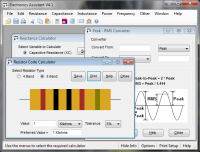# Series / Parallel Capacitor Calculator

The calculators below calculate series or parallel combinations of capacitors. Enter the capacitor value and press 'Add to Total'. Repeat until all capacitors have been entered. Press 'Clear Total' to start a new calculation.

#### Forumlae:

Capacitors in Series: (1/CTOTAL) = (1/C1) + (1/C2) + (1/C3) .....

Capacitors in parallel: CTOTAL = C1 + C2 + C3 .....

# If you found this calulator useful you should try Electronics Assistant!• All the online calculators and more in a stand-alone application
• Converts Resistor & Inductor colour codes, calculates LED series resistors, capacitance units, series / parallel resistors & capacitors, frequency, reactance & more
• Calculation of nearest preferred resistor values with a choice of 5 series from E12 to E192
• Print & save calculation results